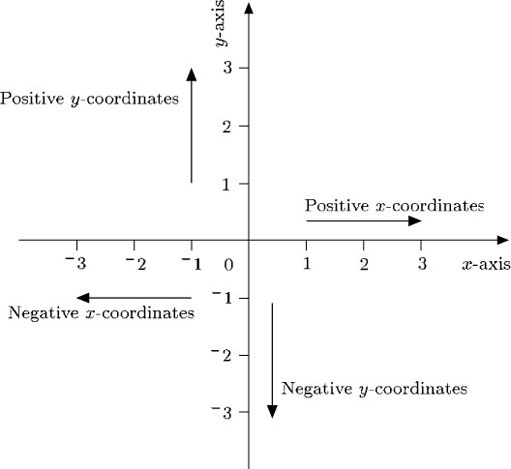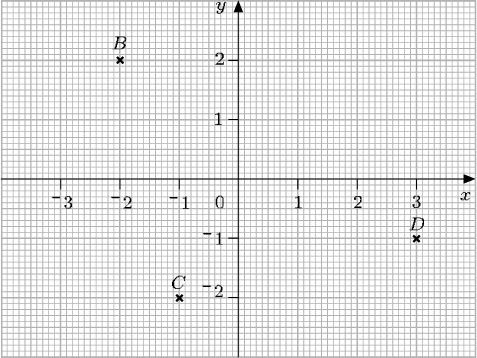Diagrams, charts and graphs

This free course is available to start right now. Review the full course description and key learning outcomes and create an account and enrol if you want a free statement of participation.

Free course

3.2 Negative coordinates

Up to now only those points with positive or zero coordinates have been considered. But the system can be made to cope with points involving negative coordinates, such as (−2, 3) or (−2, −3). Just as a number line can be extended to deal with negative numbers, the x-axis and y-axis can be extended to deal with negative coordinates.In this way, if a point is to the left of the origin, its x-coordinate is negative, and if it is below the origin, its y-coordinate is negative. The origin itself is (0, 0).

Example 10

Write down the coordinates of the points B, C and D. Remember that the x-coordinate is given first, and the y-coordinate is given second.The point B is located 2 units to the left of the origin, so the x-coordinate is −2. The point is 2 units up from the origin, so the y-coordinate is 2. The coordinates of B are written (−2, 2).

The point C is located 1 unit to the left of the origin and 2 units below it. Therefore the coordinates of C are (−1, −2).

The point D is located 3 units to the right of the origin and 1 unit below it. Therefore the coordinates of D are (3, −1).

MU120_4M5